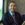# How to Calculate the VWAP

You have heard about the volume-weighted average price, or VWAP, in passing. But what is it and how do you calculate it for your swing trading pursuits? Let's find out.By Andrew Moran
Edited by Taj Schlebusch

Published October 30, 2021.

One of the commonly used investment signals for day and swing traders is the VWAP or the volume-weighted average price. The VWAP indicator is a reliable tool, even more than moving averages because it can help you determine short-term trends.

But how can you accurately apply VWAP to your trades? It is all about crunching the numbers.

### VWAP Calculation Formula

The VWAP calculation formula is pretty easy to understand: the average price of a stock weighted by the total trading volume.

Or, put another way: It is the price multiplied by the number of shares traded and then divided by the total shares traded.

Here is a formula:

Price: (High + Low + Close) / 3

VWAP: Volume x Price / Volume

Here is a VWAP calculation example example:

(10 + 15 + 13) / 3 = 12.66

If the volume is 50, it would then be: 12.66 x 50 = 633.

The cumulative volume would be, for example, 53.

The VWAP is 633 / 53 = 11.94.

### Is the VWAP Calculation the Same for All Intraday Time Frames?

It should be noted that the VWAP is not used on daily charts but rather intraday charts. Indeed, a 30-day VWAP is vastly different than a 30-minute VWAP.

That said, the VWAP would apply to any time frame: one-minute, five-minute, 15-minute, or one-hour chart.

### Look to the VWAP!

VWAP is great for all kinds of traders because it serves as a genuine representation of the true average price of the stock. While the VWAP calculation is not the same as the stock's closing price, and it does not directly impact the performance, the VWAP indicator is reliable for investors.